Function Repository Resource:

# TangentVector

Compute the tangent vector of a curve

Contributed by: Wolfram Staff (original content by Alfred Gray)
 ResourceFunction["TangentVector"][c,t] computes the tangent vector of a curve c parametrized by t.

## Details and Options

The tangent vector is a unit vector tangent to a curve or surface at a given point.

## Examples

### Basic Examples (1)

Calculate the value of the tangent vector of a curve:

 In:=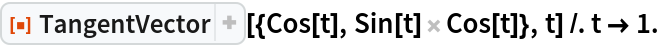Out=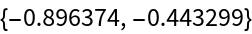### Scope (2)

Plot a set of tangent vectors:

 In:=Out=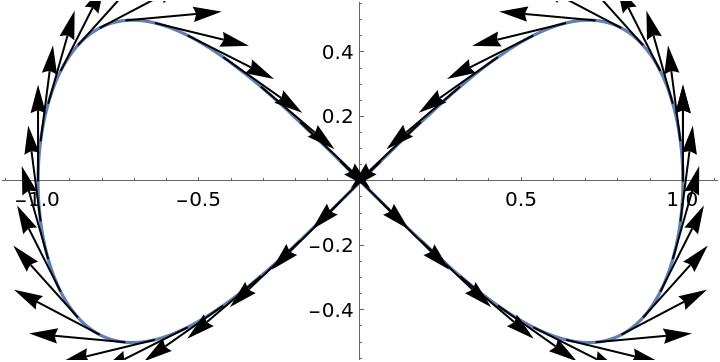The tangent vector of a figure-eight curve:

 In:=Out=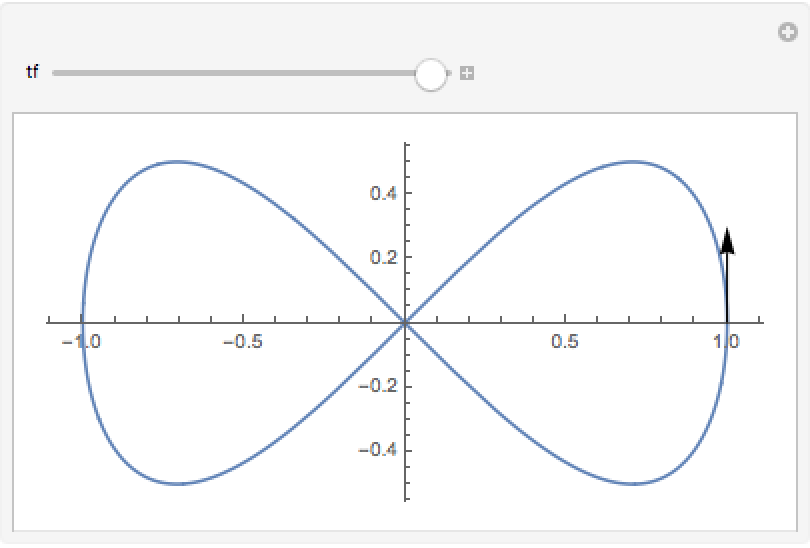### Properties and Relations (2)

Define a helix:

 In:=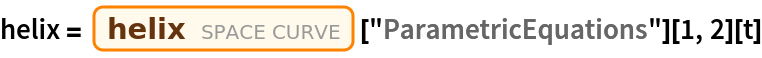Out=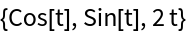The tangent developable surface of a curve is generated by the tangent vector field of the curve. It can be calculated using the resource function TangentDevelopableSurface:

 In:=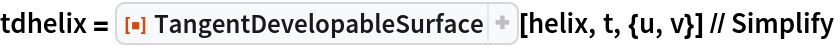Out=This is alternatively formed by adding a multiple of the tangent vector to the curve:

 In:=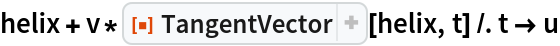Out=Plot the surface:

 In:=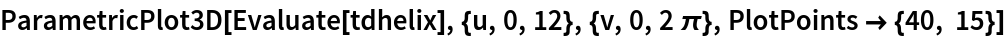Out=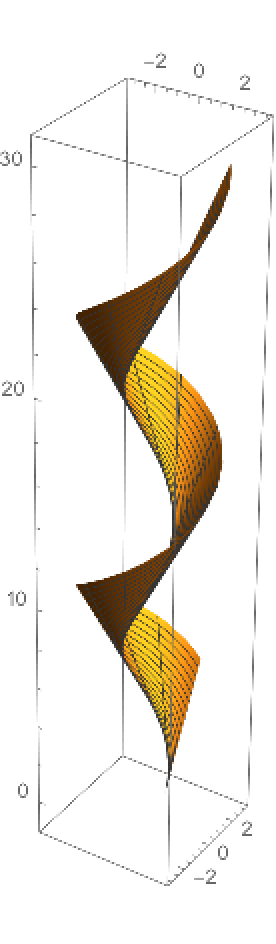A unit speed helix:

 In:=The curvature, via the resource function Curvature:

 In:=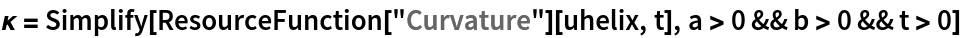Out=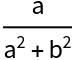The tangent vector:

 In:=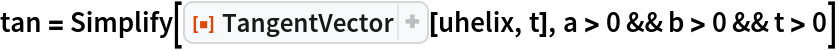Out=The derivative of the tangent vector:

 In:=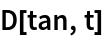Out=The normal vector, via the resource function NormalVector:

 In:=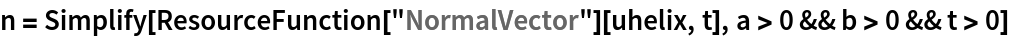Out=The curvature times the normal vector is equal to the derivative of the tangent vector:

 In:=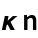Out=In relation with the Frenet-Serret system, the tangent vector is the first entry of the second List:

 In:=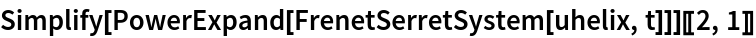Out=Enrique Zeleny

## Version History

• 1.0.0 – 28 April 2020